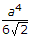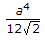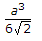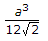# Civil Engineering - UPSC Civil Service Exam Questions - Discussion

8.

A beam of square beam is given by section 'a' is used with its diagonal horizontal. The modulus of section of the

 [A].[B].[C].[D].Explanation:

No answer description available for this question.

 Uttam said: (Feb 21, 2018) Anybody explain it, please.

 Ram said: (May 6, 2018) I think D is correct.

 Veeresh said: (Nov 7, 2018) a is diagonal means side should be a/√2. M of S =l/Z , I=bd^3/12 , Z=d/2, Hence M of S= bd^2/6 and=a^3/12√2.

 Xayaz said: (Sep 23, 2021) Z=I/Y Here i=d^4/12 & y=d/2 where d = length of diagonal=(√2)*a. So, the ans will be option C.